## SUGAR CUBES

### VOLUME OF RECTANGULAR PRISMS

Explore concepts relating to volume of rectangular prisms and how it release to multiplication and addition.

## Intentionality & Unit Overview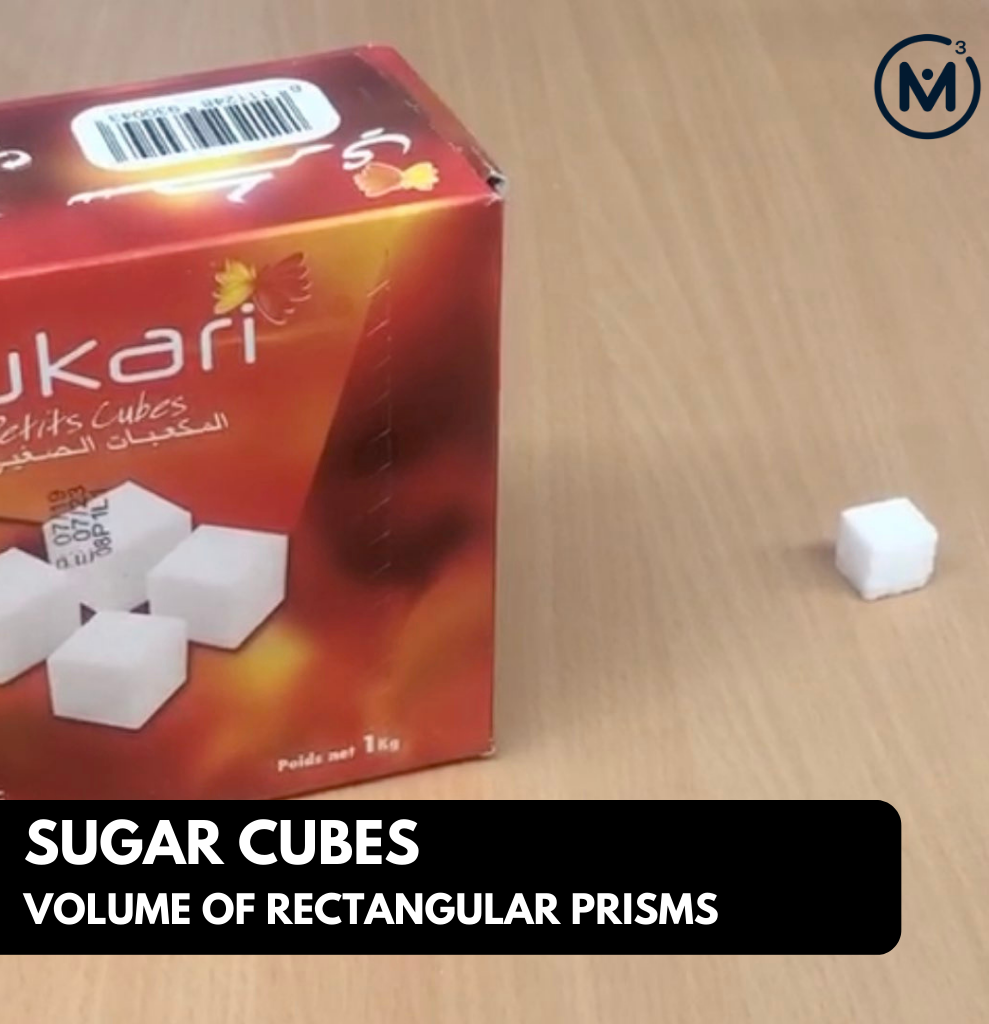### Access each lesson from this unit using the navigation links below

Students will find how many sugar cubes are in the box by finding the volume of the box using sugar cubes as the unit of measure.

## Intentionality…

In this task, students use a nonstandard unit of measure (a sugar cube) in order to determine the volume of a box of sugar. This task serves to illustrate the relationship between addition and multiplication and how the two operations can be used to determine the volume of a rectangular prism.

Some of the big ideas that will likely emerge in this task include:

• Volume is an attribute of a three-dimensional space;
• Volume can be measured by finding the total number of same-size units of volume required to fill the space without gaps or overlaps;
• The volume of a rectangular prism is related to the edge lengths;
• The volume of a rectangular prism can be found by counting the cubes in one layer and multiplying it by the number of layers;
• The volume of a rectangular prism can be determined by finding the area of the base and multiplying by the number of layers;
• The volume of a rectangular prism can be determined by multiplying length, width and height; and,
• The product remains unchanged, no matter how the numbers being multiplied are ordered (commutative property of multiplication).

## What You’ll Need…

A variety of tools for students to use to think through the problems, such as:

• Linking cubes or Omnifix cubes
• Isometric dot paper & colored pencils or markers
• Grid paper
• Whiteboards & markers
• Teaching remotely? NCTM’s Isometric Drawing Tool can be used to build and manipulate cube structures

## What Do You Notice? What Do You Wonder?

Show students the following video:

What do you notice?

What do you wonder?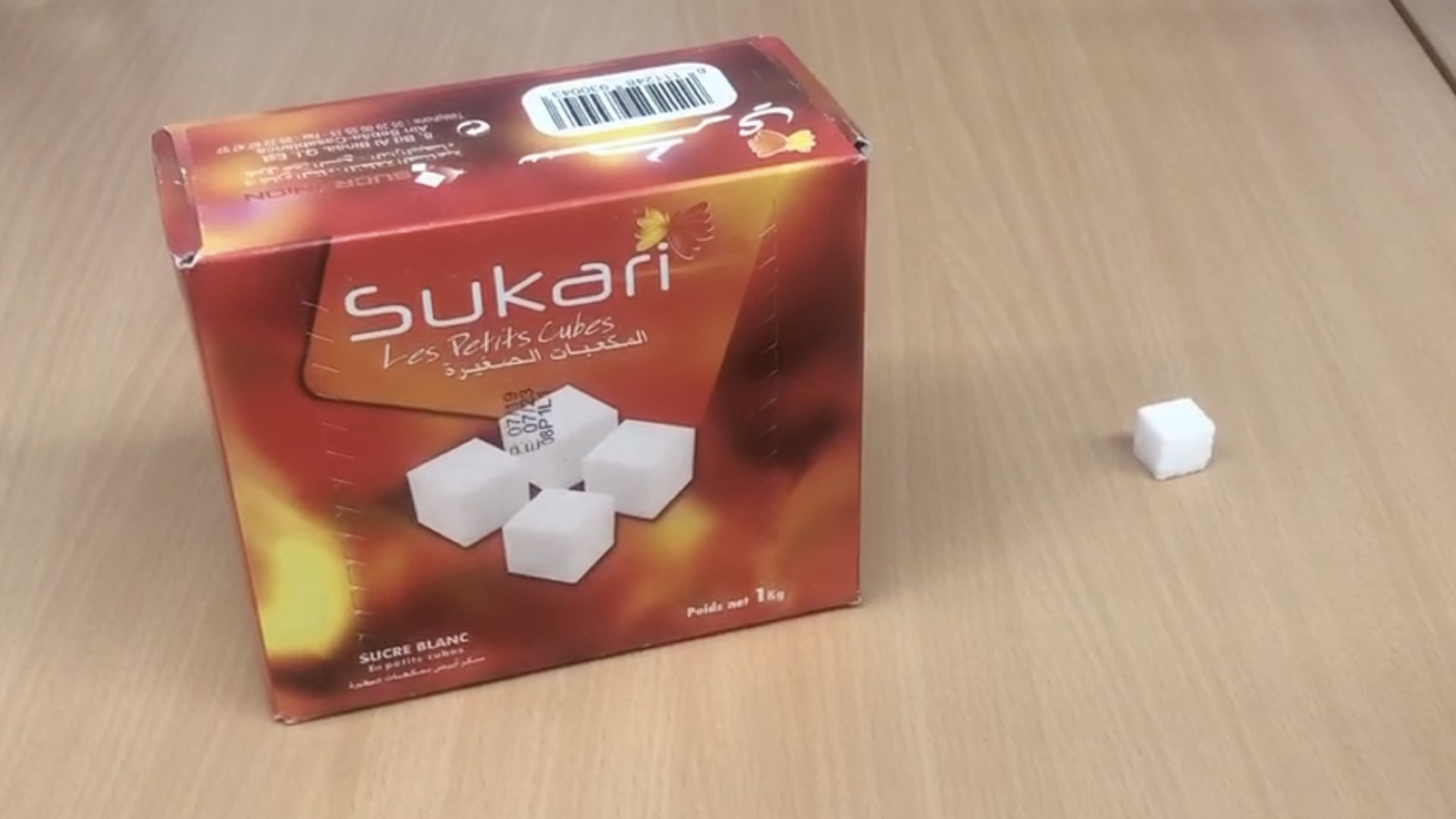Have students do a Think-Pair-Share routine:

1. Students have individual think time to jot down ideas on paper or whiteboard (minimum 1 minute). Students may benefit from watching the video a second time or having a still image from the video to refer to.
2. Students share their observations and questions with a partner.
3. Students share as a whole group, either their own noticings and wonderings, or a meaningful observation or question they heard from their partner during the share (while giving credit to their partner). All contributions are acknowledged and recorded on an anchor chart on the board.

Possible points that may come up include:

• I notice there’s a box of sugar with a single cube next to it.
• I wonder how many sugar cubes are in the box?
• I wonder how much the box costs?
• I wonder how much a single cube costs?
• I notice the box hasn’t been opened.
• I wonder how many calories are in the box?
• I wonder what the dimensions of the box are?
• I wonder how much the box weighs?
• I wonder how much a single cube weighs?

Some wonderings can be answered immediately and crossed off the list:

• The box weighs 1kg.
• The box costs \$1.95.

Spending time to acknowledge and address specific thoughts that students shared, whether a notice or a wonder, is crucial to building a culture in your classroom where students know that their voice is being valued and thus encourages them to continue sharing their thoughts and opinions later in this lesson and in future lessons.

## Estimation: Prompt

Once student noticings and wonderings have been acknowledged and noted, the class can settle on a first question to explore:

How many sugar cubes are in the box?

Make an estimate.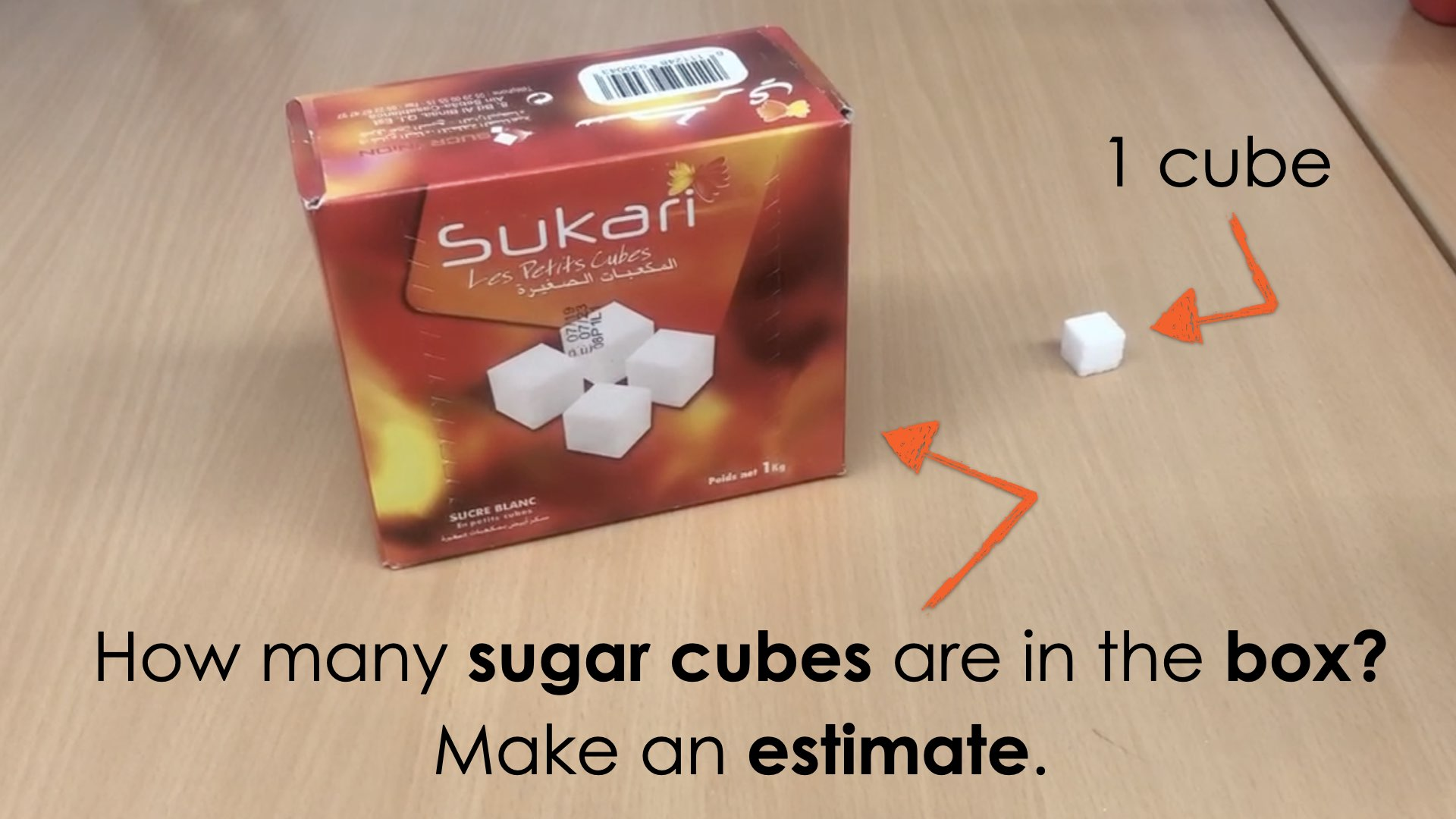Students begin by making an estimate before they are provided with all the information they need to answer the question, thus requiring them to consider what would be a reasonable number of cubes.

Encouraging students to begin by considering estimates that are “too high” and “too low” will provide a range within which more likely estimates can be framed. It can also provide students who may be reluctant to share with a safe entry point. Students can then make a “reasonable estimate”, which they will have a chance to revise.

Be sure not to skip over asking students to make an estimate using only their spatial reasoning skills as this is a very important step in the Curiosity Path. Providing students an opportunity to make an estimate and try to articulate their thinking with their peers provides a very low floor opportunity for them to not only better understand the context, but to also begin nudging them to think about what will be important to make their estimate more precise as we continue through the lesson.

Students should be given an opportunity to share their estimates at this point, but refrain from sharing their rationale just yet in order to give everyone a chance to develop their own thinking.

Have students turn to a partner and generate questions they could ask that would provide them with information they could use to answer the question. Ask students how they would use the information to answer the question.

Prompt:

What information would you need to answer the question?

How would you use that information?

## Estimation: Partial Reveal & Update

Reveal to the students the image of a single layer of sugar cubes: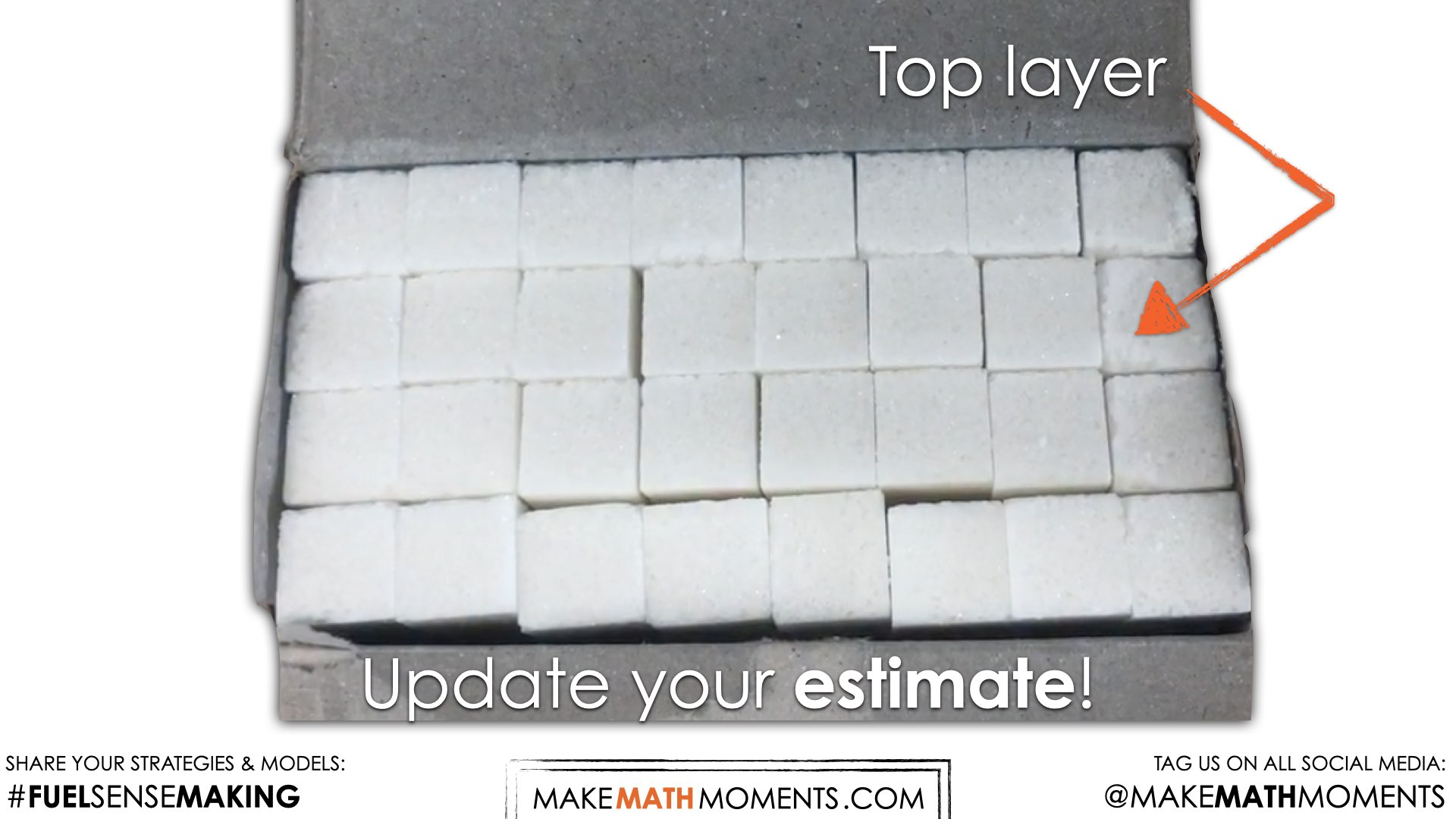At this point, students may wish to update their estimates. Provide them with time to do so before revealing the next image. A simple prompt might be:

You’ve seen how many cubes are in a single layer. Does this affect your estimate? Would you say your estimate is still reasonable, or will you revise it?

Provide students with time to revise their estimates.

## While Students are Revising Their Estimates

Listen and observe as students revise their estimates. Are students aware that the layered structure of a rectangular prism allows them to think additively or use multiplication?

How are students approaching the problem? What strategies and mathematical models are they leveraging? Are they:

• Counting cubes one by one?
• Making use of the additive nature of volume?
• Using the layered structure in a rectangular prism to think about volume using multiplication?
• Using area models to find products?
• Using an algorithm?

This is an opportunity to make note of where students are developmentally. Prompt students to explain their thinking and how they plan to use their model/strategy to determine the total number of sugar cubes in the box.

## Crafting A Productive Struggle: Prompt

Proceed to reveal the number of layers by showing the following image: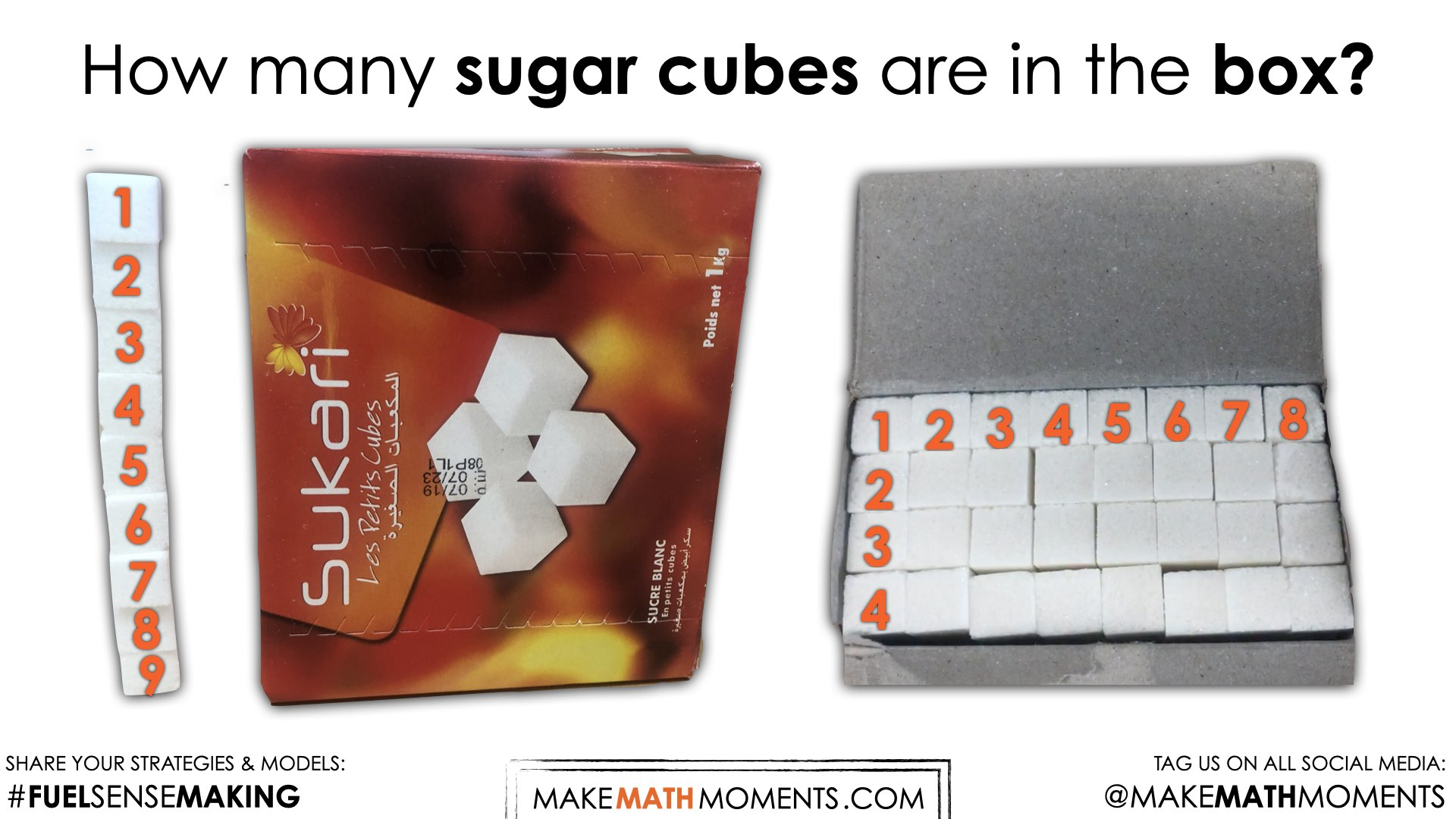To begin the sense making portion or the lesson, provide students with the following prompt:

There are 9 layers of sugar cubes in the box. If each layer has 4 rows of 8 cubes, how many sugar cubes are in the box?

Use a mathematical model of your choice to convince your mathematical community.

Be sure to explicitly state that calculators are not to be used to determine the number of sugar cubes in the box. However, do consider making the following tools available to students without explicitly directing students to use them:

• Linking cubes or Omnifix cubes
• Isometric dot paper & colored pencils or markers
• Grid paper
• Whiteboards & markers
• Teaching remotely? NCTM’s Isometric Drawing Tool can be used to build and manipulate cube structures

Remind students to refrain from shouting out answers, and be sure to emphasize the importance of creating a viable argument – a model that can be used to convince others.

## While Students Are Productively Struggling…

Monitor student thinking by circulating around the room and listening to the mathematical discourse. Select and sequence some of the student solution strategies and ask a student from the selected groups to share with the class from:

• most accessible to least accessible solution strategies and representations;
• most common/frequent to least common/frequent strategies and representations; or,
• choose another approach to selecting and sequencing student work.

The tools and representations you might see students using to convince their peers and/or the teacher include, but are not limited to:

• Concrete manipulatives to directly model the rectangular prism
• Mix of multiplicative and additive strategies
• Array or area model for the base with second model for layers
• Flexible use of the standard multiplication algorithm

Common misconceptions you may consider noting include:

• Confusing volume and surface area
• Using the same dimension twice in calculations

During the consolidation phase, prior to asking students to share their own strategies and reasoning, ask the class if they can discern how the students whose work is being shared were thinking about the problem.

A possible prompt might be:

Can you tell how this student/group was thinking about the problem?

This encourages all students to consider alternative viewpoints and begin to seek connections between representations. It helps set up the mindset of “listening to understand” when students share their work.

Have students share their strategies and reasoning for how to represent the number of sugar cubes in the box. Ask them to convince you and their peers that their answer is correct by sharing mathematical models.

Discuss their strategies and elicit student thinking during your consolidation to build off of their current prior knowledge and understanding rather than “fixing” or “funnelling” student thinking to a strategy and/or model that does not connect to their strategy and/or approach.

## Student Approach #1: Concrete Representation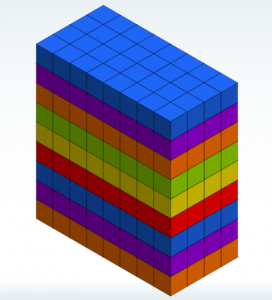I built one layer by looking at the dimensions on the picture. It was four rows of eight cubes. Then I saw that there were 9 layers. Each layer had 32 cubes, so I added the layers together and got 288 cubes.

Facilitator’s note:

Different concrete representations may provide an opportunity to reinforce concepts related to multiplication, such as the commutative property and the associative property, as the rectangular prisms can be held at different angles.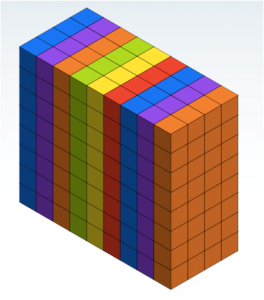## Student Approach #2: Direct Modeling and Area Model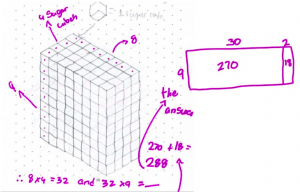We built the structure and drew it on the dot paper. We saw that one layer was 4 x 8 which is 32. There were 9 layers, so we had to multiply 32 x 9. We decomposed 32 into 30 and 2. 30 x 9 is 270, and 2 x 9 is 18, so the answer is 288 cubes.

## Student Approach #3: Array and Algorithm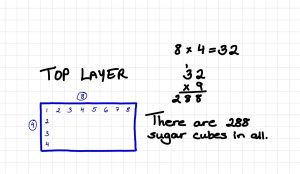I saw that the top layer was 4 x 8, which is 32 cubes. I multiplied 32 x 9 because there are 9 layers. So, in all, there are 288 sugar cubes in the box.

## Reveal

Consider sharing the following reveal video with your students:

Alternatively, you could share the reveal image: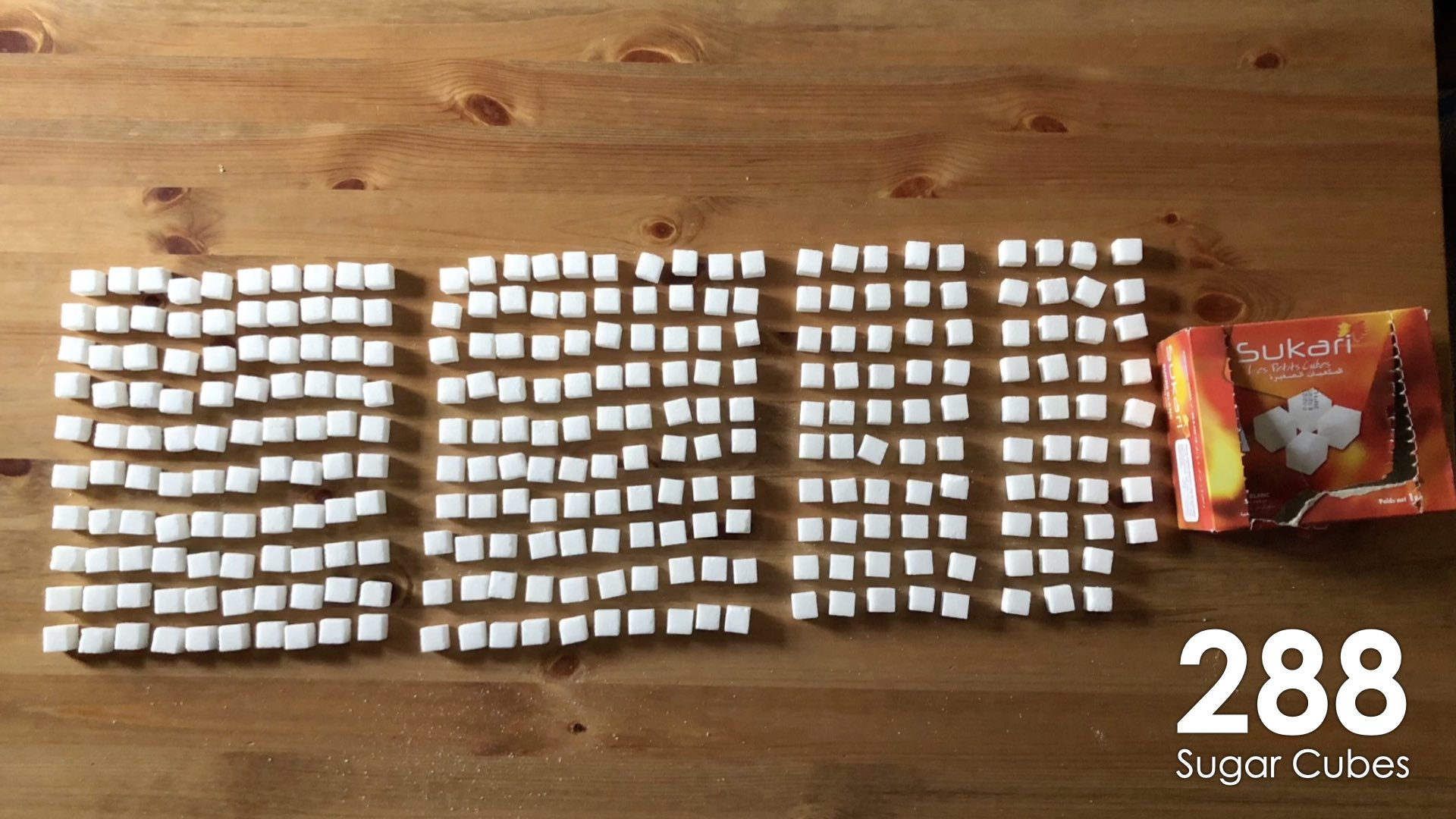## Consolidation

During the consolidation, be sure to gather student work samples or make note of student approaches while you were monitoring as students work productively struggling.

Facilitate a discussion by referencing some of the student approaches including their strategies, tools and representations.

If a/some students noticed that there was a relationship between the dimensions of the prism and its volume (whether it was on their own or from your purposeful questioning), be sure to ask one of those students to share their discovery with the group.

During consolidation, highlight how students went about finding the number of sugar cubes in the box. Did they count each cube individually? Did they find the number of cubes in the base layer, then repeatedly add to account for the number of layers?

As you are discussing student solutions, there is the opportunity to highlight the connection between the volume, the area of the base, and the length of the edges.

Prompt:

What connections do you see between the dimensions (length, width, height) of the sugar box and its volume?

Consider providing students with a word bank that includes the following words:

cubes – volume – layer – dimensions – base – length – width – height – multiply

Conduct a Think-Pair-Share where students first have a moment to consider the question on their own, then turn and talk to a partner, then share as a whole group. Students will likely notice that multiplying the length, width and height of the rectangular prism will yield its volume.

Clearly communicate both orally and in written form the information that was known and unknown in this problem.

Given:

The total number cubes in one layer, which can also be called the base (32, or 8 x 4)

The total number layers (9)

Revealed:

The number of sugar cubes in the box (288)

Reinforce that this scenario can be represented as:

32 cubes in a single layer x 9 layers = 288 cubes

Ask students to consider the following scenarios:

If the sugar box had a top layer of 4 x 9, with a total of 8 layers, how many sugar cubes would there be?

If the sugar box had a top layer of 8 x 9, with a total of 4 layers, how many sugar cubes would there be?

It may be worthwhile to consider developing a shared method for recording the dimensions of a rectangular prism, with the length and width of the base in parenthese, followed by the height, as follows (length x width) x height.

## Reflect and Consolidation Prompts

Consider asking students to complete the following consolidation prompts independently.

Making Connections

1. If the sugar box had a top layer of 4 x 9, with a total of 8 layers, how many sugar cubes would there be?
2. If the sugar box had a top layer of 8 x 9, with a total of 4 layers, how many sugar cubes would there be?

What Is The Same? What Is Different?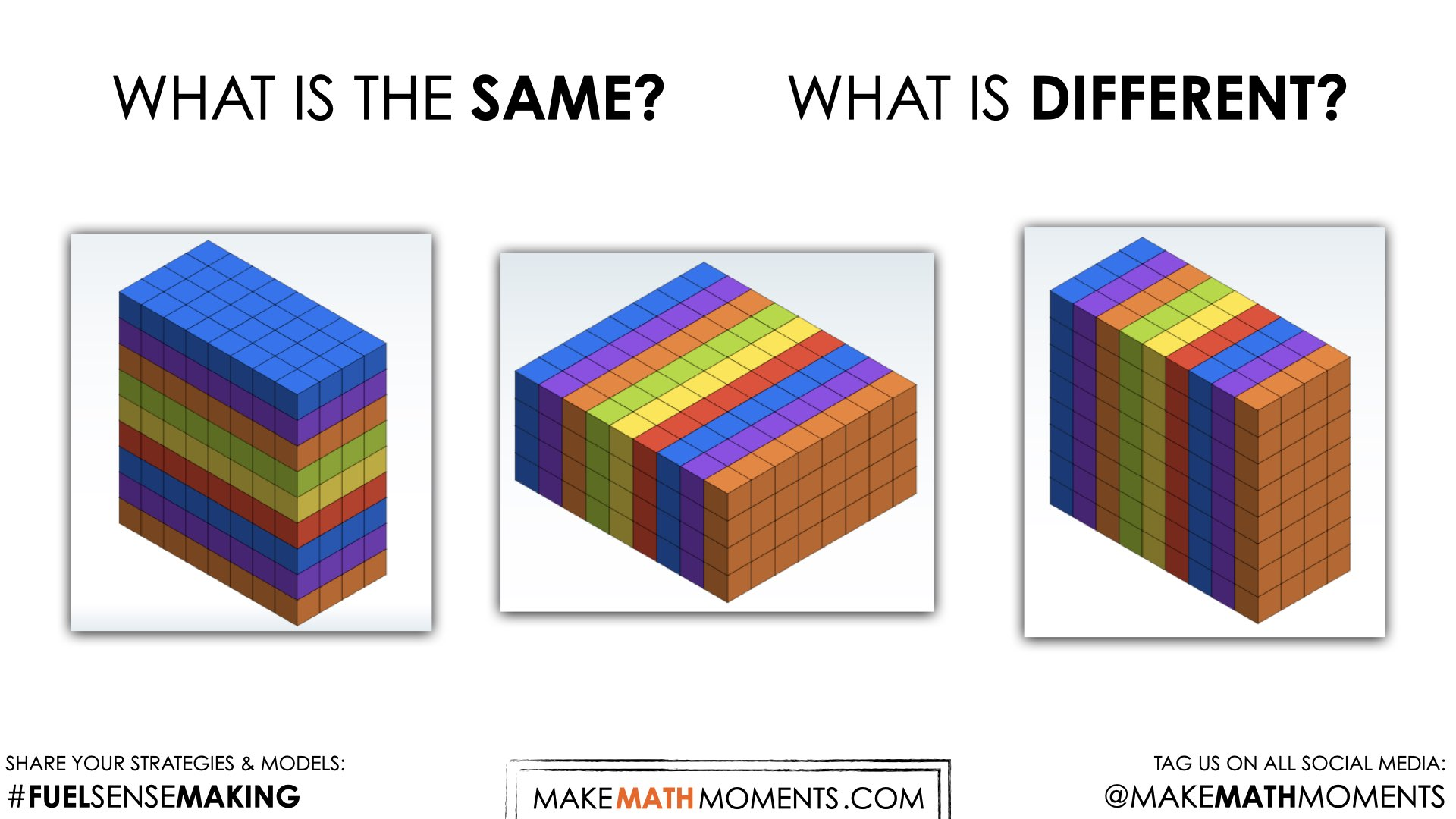Making Connections

What connections do you see between the dimensions (length, width, height) of the sugar box and its volume?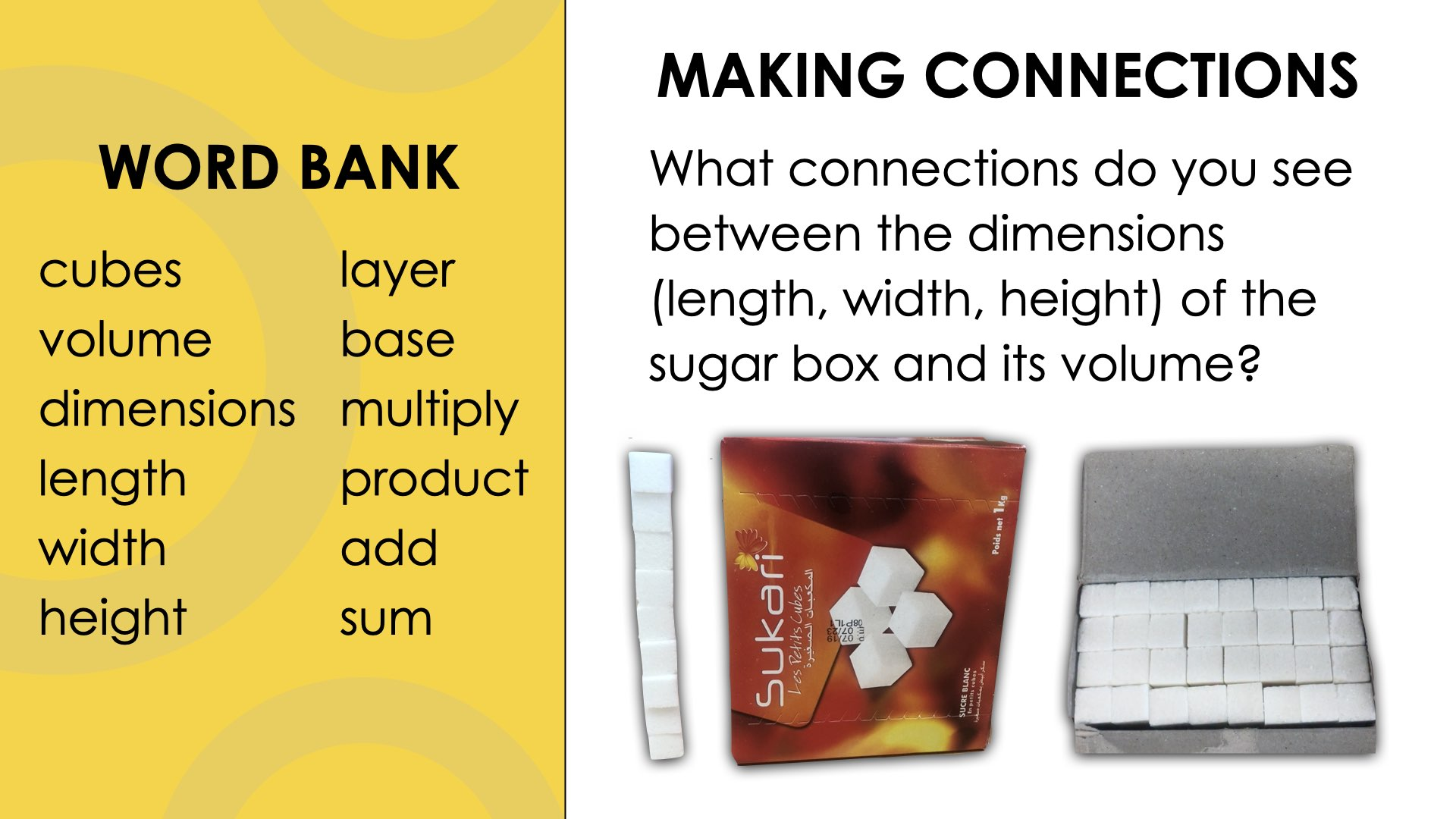Reflect

What might a general rule be for finding the volume of a rectangular prism?

Consolidation Prompt:

Consider the following scenarios:

1. A box of dice has 5 rows of 6 dice in the bottom layer. The box holds 8 layers of dice. How many dice does the box hold?
2. A moving truck holds 8 rows of 12 same-size boxes in the bottom layer. The boxes are stacked 6 layers high. How many boxes does the truck hold?

For both scenarios above:

1. What information is given?
2. What information are you trying to reveal?
3. Model both scenarios and solve.

## Educator Discussion Area

Login/Join to access the entire Teacher Guide, downloadable slide decks and printable handouts for this lesson and all problem based units.

## Explore Our 60+ Problem Based Units

This Make Math Moments Lesson was designed to spark curiosity for a multi-day unit of study with built in purposeful practice, number talks and extensions to elicit and emerge strategies and mathematical models.

Dig into our other units of study and view by concept continuum, grade or topic!